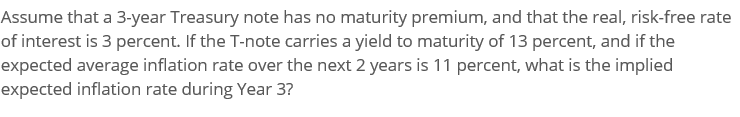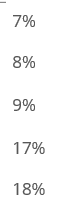# (Solved): Assume That A 3-year Treasury Note Has No Maturity Premium, And That The Real, Risk-free Rate Of Int...please show work in written form (not excel) thank you.

Assume that a 3-year Treasury note has no maturity premium, and that the real, risk-free rate of interest is 3 percent. If the T-note carries a yield to maturity of 13 percent, and if the expected average inflation rate over the next 2 years is 11 percent, what is the implied expected inflation rate during Year 3? 7% 8% 9% 17% 18%

We have an Answer from Expert